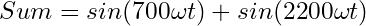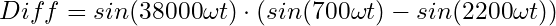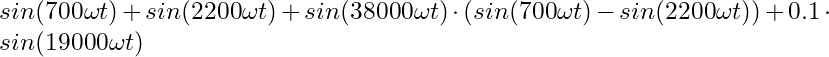# Generate a stereo-FM multiplex waveform with Python and AWG

Generating more complex arbitrary waveform files for modern test equipment doesn’t have to be difficult. For a recent project, I needed a stereo-FM multiplex (MPX) signal containing two different tones in the left and right stereo audio channels. This article is going to show how to generate such a MPX signal for Siglent SGD-series arbitrary waveform generators with Python and PyVISA.

UPDATE: There also is a German version of this article available on my German blog: Stereo-Multiplexsignal mit einem Funktionsgenerator und Python erzeugen

#### General Overview

The way stereo-FM works is both simple and sophisticated at the same time. First, sum and a difference signals of the left and right audio channels are created. The sum signal ensures backward compatibility with legacy mono FM receivers. A double sideband signal centered around 38 kHz is generated using the difference signal and a 38 kHz carrier. Lastly, a 19 kHz pilot tone, that is phase coherent to the 38 kHz carrier used in the previous step, is added to the previous 2 signals with a relative amplitude of 10 %. All three signals are then transmitted together using FM modulation. This is a short and simplified explanation. For a better explanation, feel free to watch my YouTube video on the theory of FM stereo multiplexing .

My goal was to generate a valid MPX signal containing a 700 Hz tone in the left audio channel and a 2200 Hz tone in the right audio channel. On the hardware side I settled on using my Siglent SDG1032X arbitrary waveform generator (AWG). On the software side, I decided on using Python with PyVISA and NI-VISA. The latter two offer a very convenient way of communicating with the AWG via Ethernet.

#### Waveform Math

The first step was to figure out how to generate the necessary datapoints for the MPX signal. In order to achieve this, equations describing all components of the final signal in a mathematical form needed to be formulated. For the sum signal, the DSB modulated difference signal and the 19 kHz pilot tone, the equations are as follows:

Equation for the sum (L+R) signal:Equation for the difference (L-R) double sideband signal with 38 kHz carrier:Equation for the 19 kHz pilot tone:Where ω in this case is a normalization factor to convert the frequencies from cycles per second to radians per sample. Time – expressed as sample number – is represented as t. For the Python / SDG combination, the normalization factor ω is π divided by twice the sample rate. Since the sum, DSB modulated difference and pilot tone signal are simply added together, the overall equation for the desired MPX signal can be written as follows:Note that this equation will return a maximum value of 2.1 and a minimum value of -2.1. So when scaling this equation to the desired maximum amplitude, the amplitude values needs to be multiplied by the inverse of 2.1, or multiplied by about 0.47.

#### Python implementation

The entire Python code, along with other Python / PyVISA example scripts, is available from my SIGLENT GitHub repository .

The Python code needs to create an array of 16384 points filled with waveform data according to the aforementioned equations. While the following code snippet may not be the prettiest, it works:

# Create an empty array with 16384 points WAVE = np.arange(0, 0xfffe, 1);

 # Sample Rate in S/s SAMPLE_RATE = 1638400 # Calculate factor for normalized frequency F_FACTOR = (np.pi/(2*SAMPLE_RATE)) # Fill the waveform array with data for n in range(len(WAVE)): 

  # Amplitude (MAX 32767 on SDG1032X)   Amplitude = 32767   WAVE[n] = 0.47*Amplitude*(np.sin(700*F_FACTOR*n)+np.sin(2200*F_FACTOR*n)+0.1*np.sin(19000*F_FACTOR*n)+np.sin(38000*F_FACTOR*n)*(np.sin(700*F_FACTOR*n)-np.sin(2200*F_FACTOR*n)))

The generated waveform data is then sent to the SDG using the write_binary_values of the PyVISA package and the necessary SCPI commands. This of course requires the PyVISA package and the NI-VISA API to be installed and that a connection to the device has been established.

# Write Waveform to Device # Note: byte order = little-endian! device.write_binary_values('C1:WVDT WVNM,STEREO_MPX,FREQ,100.0,TYPE,8,AMPL,1.0,OFST,0.0,PHASE,0.0,WAVEDATA,', WAVE, datatype='i', is_big_endian=False)

#### Results

After executing the Python code and sending the generated waveform to the SDG1032X, the MPX signal is generated as intended. Since only two distinct tones are being generated, the corresponding spectral components and their relative amplitudes can clearly be observed if viewed in the frequency domain:

And just for completeness, here’s the same signal in the time domain:

#### Conclusions

In essence, it could be said that as long as an equation for a waveform can be formulated, it is rather simple to generate arbitrary waveform files to ones heart’s content. Of course there are some limitations to this. In this case mostly the relatively small 16384 possible points. Nonetheless, the framework of the Python code provided offers great versatility for the quick implementation of arbitrary waveforms. Just adapt the line beginning with “WAVE[n] =” to implement your own waveforms. The GitHb repository contains a few more simplistic examples as well.

 BalticLab (2016): Stereo Multiplexing for FM Transmission | Theory

 AI5GW (2022): Python code examples for SIGLENT equipment